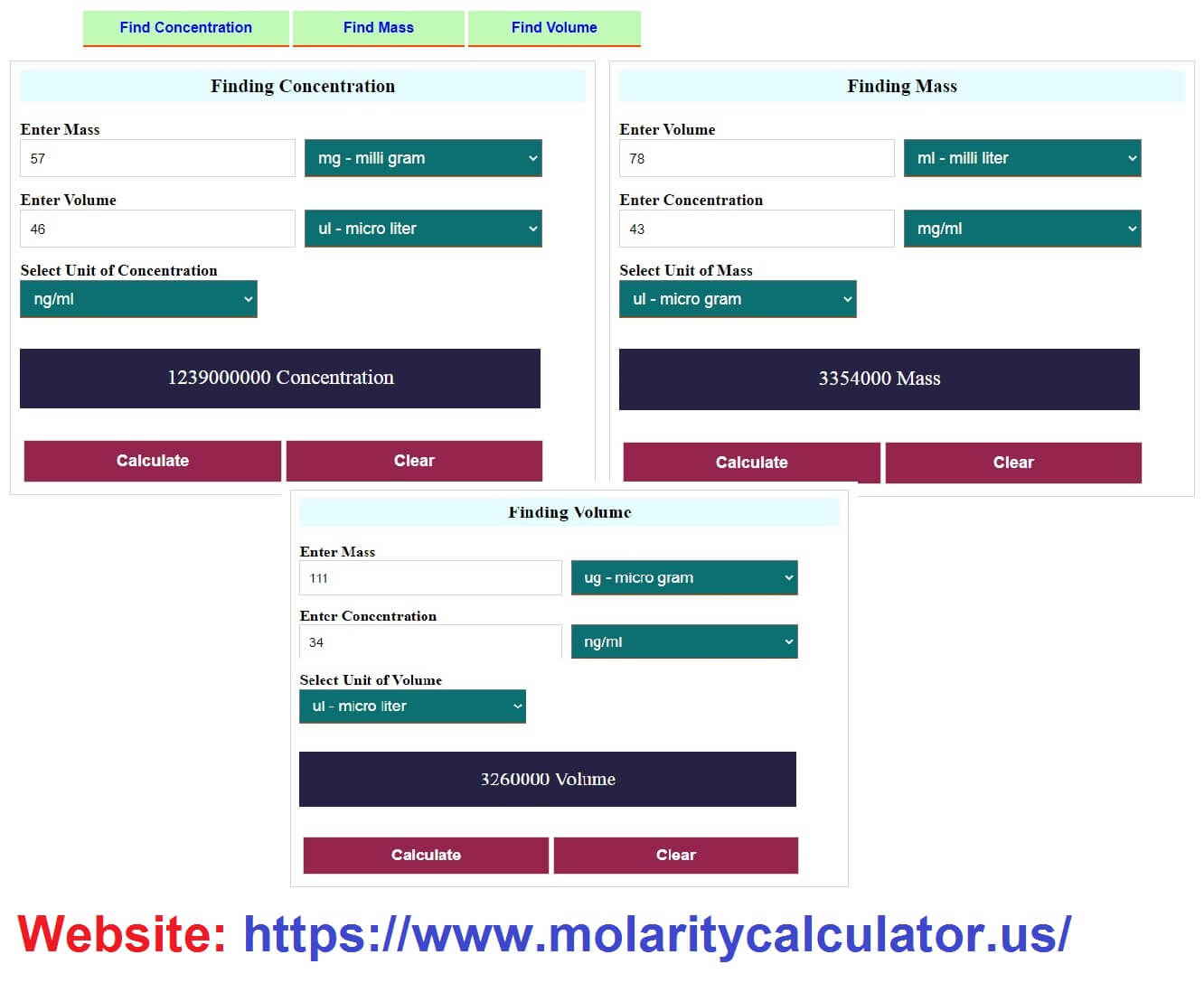## Concentration Calculator

Finding Concentration

Enter Mass

Enter Volume

Select Unit of Concentration

Your Result Is Here

Finding Mass

Enter Volume

Enter Concentration

Select Unit of Mass

Your Result Is Here

Finding Volume

Enter Mass

Enter Concentration

Select Unit of Volume

Your Result Is Here

# Molar concentration How To Find Concentration

It is the ratio of solute in a solution or amount of a matter in a defined space.
Concentration calculates from (Mass & Volume).

concentration = mass / volume

### What is Molar Concentration Calculator?

Concentration Calculator is a tool for calculating the concentration from (Mass & Volume), you can give the numbers and select the units (volume, mass) to calculate.

#### How This Calculator works?

In Calculate Concentration there are 3 things (Finding Mass, Finding Volume & Finding Concentration).
If the values of a unit are not known then you can get the value in its respected section.

##### Finding Mass How To Calculate Mass

Finding the (Mass) from (Concentration & Volume). When the (Mass) is unknown, you can use this section.
(Enter Value in Concentration textbox and Select the Unit of Concentration)
(Enter Value in Volume textbox and Select the Unit of Volume) to Calculate the (Mass).

mass = (volume * concentration)
exp: 15 * 20 = 300 milligrams of Mass

###### Finding Volume How To Find Volume

Here finding the (Volume) from (Concentration & Mass). When the (Volume) is unknown, you can use this.
(Enter Value in Concentration textbox and Select the Unit of Concentration)
(Enter Value in Mass textbox and Select the Unit of Mass) to Calculate the (Volume).

volume = (mass / concentration)
exp: 30 / 20 = 1.5 milliliters Volume

Concentration Formula Finding Concentration

Find the (Concentration) from (Volume & Mass). When the (Concentration) is unknown, you can use.
(Enter Value in Volume textbox and Select the Unit of Volume)
(Enter Value in Mass textbox and Select the Unit of Mass) to Calculate the (Concentration).

concentration = (mass / volume)
exp: 45 / 60 = 0.75 milligram/milliliter Concentration